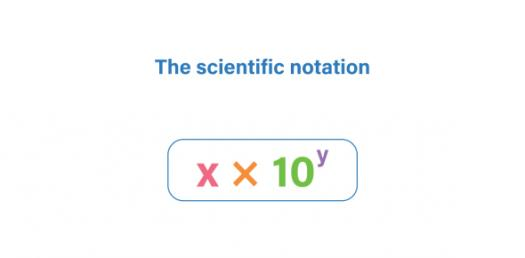# The Scientific Notation Practice Quiz- I

8 Questions | Attempts: 1754SettingsPowers of ten and scientific notation

• 1.
Write the number 4,500,000 in scientific notation.
• A.

4.5 x 10^6

• B.

45 x 10^5

• C.

4.5 x 10^-6

• D.

45,000

• 2.
Change to standard notation. 6.5 X 10^4
• 3.
Change to scientific notation..06 X 10^-3
• 4.
Check any of the following that are correctly in scientific notation:
• A.

93 x 10^2

• B.

0.93 x 10^2

• C.

9.3 x 10^2

• D.

4 x 10^13

• E.

9.4 x 10^-12

• 5.
If you are trying to convert a very small decimal into scientific notation the exponent of the 10 will always be ________________. (positive or negative)
• 6.
If you are trying to convert a very large number into scientific notation the exponent of the 10 will always be __________________ (positive or negative).
• 7.
Convert 5,345 into scientific notation. Use the form: #.###x10^# with NO SPACES IN BETWEEN.
• 8.
Convert 0.000076 to scientific notation. Use the form: #.#x10^# with NO SPACES IN BETWEEN.

## Related Topics

×

Wait!
Here's an interesting quiz for you.